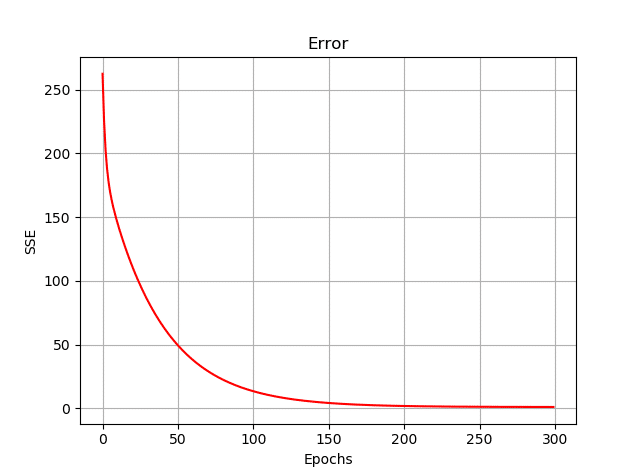# Linear Regression from Scratch using Numpy

##### So, if we consider our synthetic data to be a bunch of scalars, and 1-Dimensional, this is the simple ANN structure that we could be interested in building from scratch!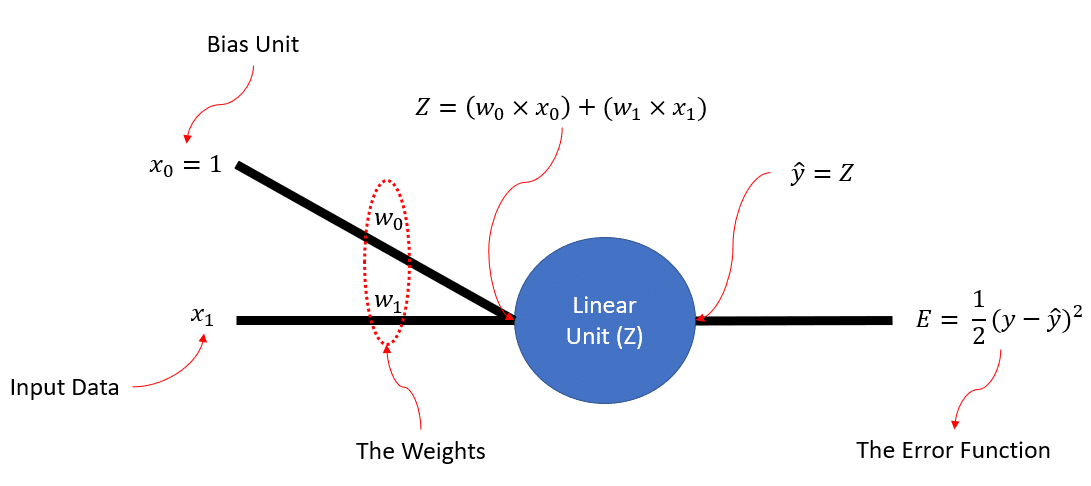##### I am sure that as a Neural Network enthusiast, you are familiar with the idea of the Linear() function and the sum squared error function. We need to use them during the forward-pass phase of training our ANN. Before showing you the code, let me refresh your memory on the math. So, the Linear() function can be defined as:##### And the sum squared error for ground-truthand prediction, can be defined as:• ##### The derivative of the error,, w.r.t our output,:The derivative of our output,, w.r.t:The derivative of, w.r.t.and:

## Initializing our Weights

##### This random line can be visualized using these weights as its slope and intercept and of course by plugging in our data,. Let’s see our does this line fit our data: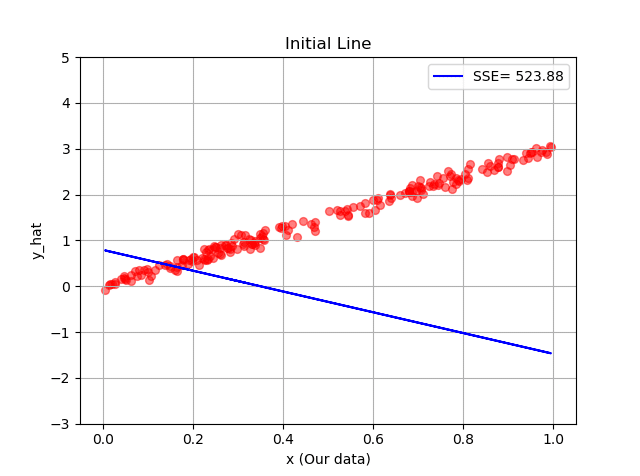## Training our Neural Network

##### During the backpropagation, we compute the gradients of our error function,, w.r.t our weights, and update the weights. We have seen the derivations of the gradients above, and just as a reminder, the update rules for our weights are:Enjoy the code now: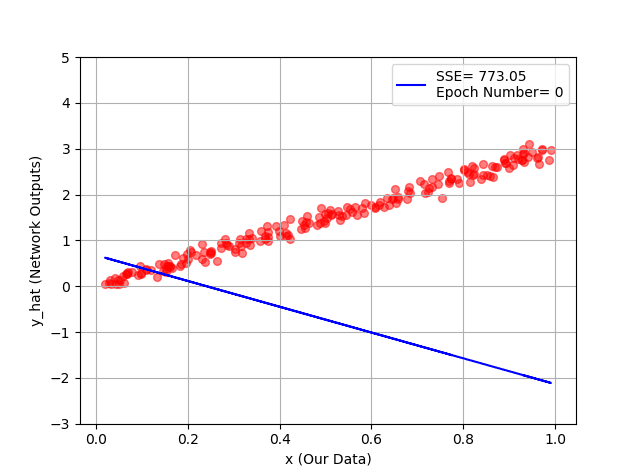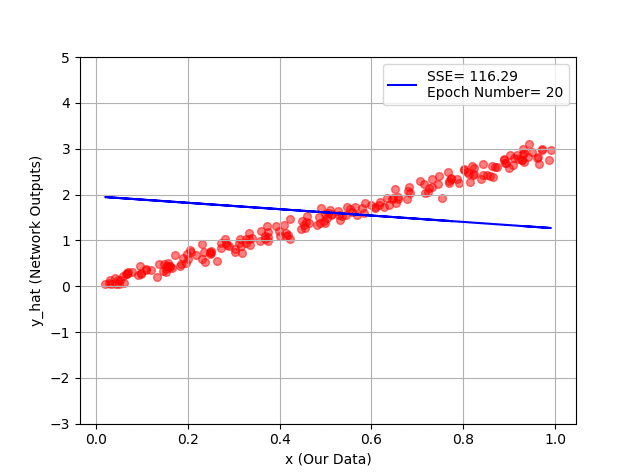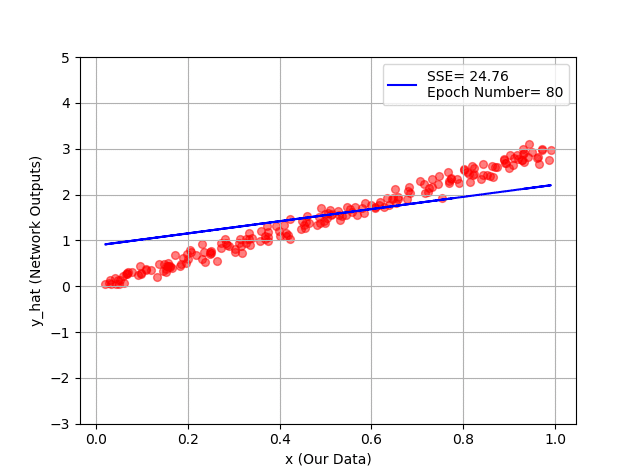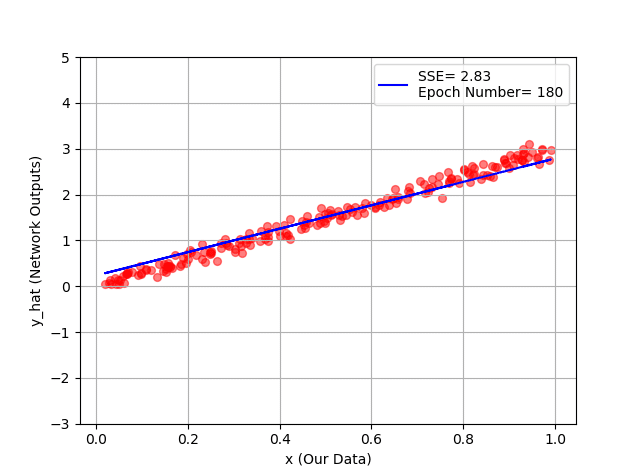##### And the error function (i.e., sum squared error) is plotted down below, during our training. Below is the code for this visualisation: